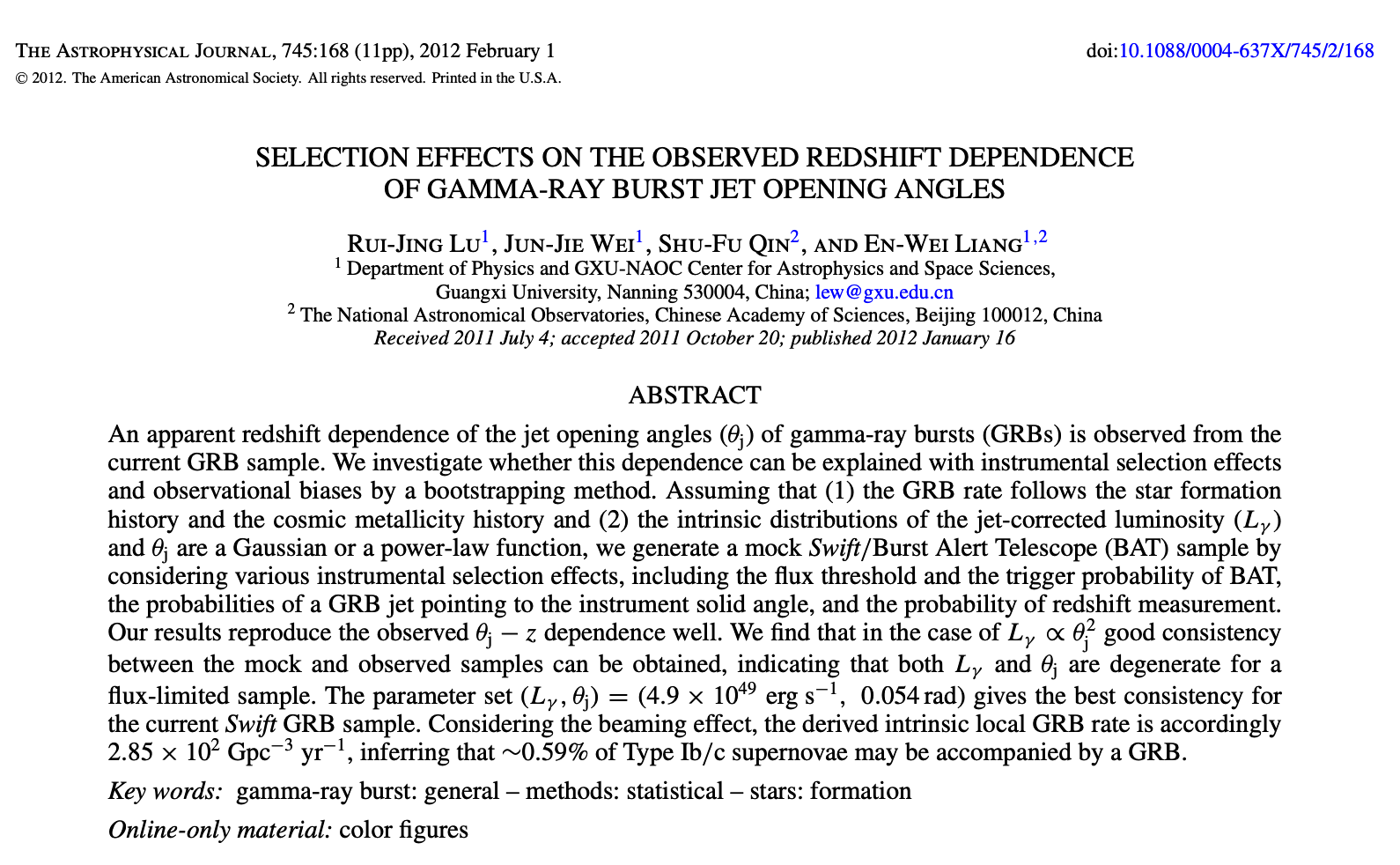What a nice day.

Tiancheng Luan, 21111162

# 2021.9.13

## Requirements:

1. Find an abstract of a research paper, and analyze its structure, contents and stock phrases.

## Results:This paper can be found at: DOI:10.1088/0004-637X/745/2/168

## Analysis:

### The structure and contents:

1. Brief Introduction and motivation of this work
First they introduce the observation fact that there is dependence between redshift and Gamma-ray burst (GRB) jet opening angle ($\theta_j$), and they want to use the instrumental selection effect and observation bias through bootstrap procedure to elaborate this dependence.
2. The pre-assumption and methodology of this work
They assume
• GRB rate follow the star formation and metallicity history.
• Intrinsic distributions of $L_{\gamma}$ and $\theta_j$ are a Gaussian or a power-law function.
• They use bootstrap program to generate mock Swift GRB sample, and set specific threshold and trigger probability as criteria whether the mock GRB can be observed by the telescope.
3. Report of results
• Their results reproduce the observed $\theta_j− z$ dependence well.
• They find when $L_{\gamma} \propto \theta_j^2$, the mock can be well consistent with observation.
• They find the best observe-fitted parameters: $(L_{\gamma}, \theta_j)=(4.9\times10^{49}erg \,s^{-1}, 0.054\,rad)$.
• They derive the intrinsic local GRB rate is accordingly $R_{GRB}=2.85 \times 10^2\,Gpc^{-3}\, yr^{-1}$, this implies about $0.59\%$ of Type Ib/c supernova may be accompanied by a GRB.

### The stock phrases:

- ... whether sth. can be explained with ...
- The form of the parameter group, it should be put in brackets.
- The unit should not be *italic*.
- well, indicating, inferring